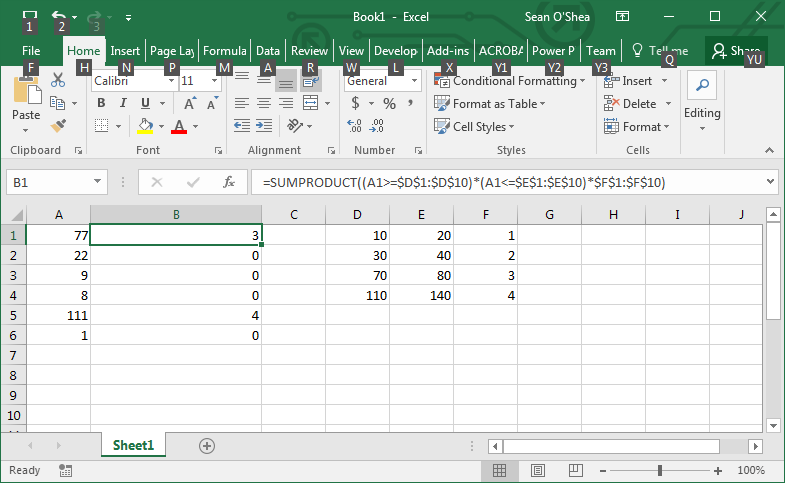Search

# Excel formula to find if list of numbers falls in range of numbers listed in two columns

You can use the SUMPRODUCT formula to check and see if a list of numbers in one column falls within a range of numbers given in two other columns. This can come in handy, if for example, you have a list of Bates numbers and want to know if they all fall with PRODBEG and PRODEND ranges in a document production. In this example, we have a list of numbers in column A, and ranges of produced documents in columns D and E. Column F simply contains a reference to be returned by the formula. So as you can see this formula:

=SUMPRODUCT((A1>=\$D\$1:\$D\$10)*(A1<=\$E\$1:\$E\$10)*\$F\$1:\$F\$10). . . finds when the value in column A is between the values in columns D and E, and then when this is true gives you the number in column F. Thanks to CRondao for posting this solution at www.stackoverflow.com.